# CBSE Class 11 Mathematics Trigonometric Function Notes

Download CBSE Class 11 Mathematics Trigonometric Function Notes in PDF format. All Revision notes for Class 11 Mathematics have been designed as per the latest syllabus and updated chapters given in your textbook for Mathematics in Class 11. Our teachers have designed these concept notes for the benefit of Class 11 students. You should use these chapter wise notes for revision on daily basis. These study notes can also be used for learning each chapter and its important and difficult topics or revision just before your exams to help you get better scores in upcoming examinations, You can also use Printable notes for Class 11 Mathematics for faster revision of difficult topics and get higher rank. After reading these notes also refer to MCQ questions for Class 11 Mathematics given on studiestoday

## Revision Notes for Class 11 Mathematics Chapter 3 Trigonometric Functions

Class 11 Mathematics students should refer to the following concepts and notes for Chapter 3 Trigonometric Functions in Class 11. These exam notes for Class 11 Mathematics will be very useful for upcoming class tests and examinations and help you to score good marks

### Chapter 3 Trigonometric Functions Notes Class 11 Mathematics

XI: Maths

Chapter-3: Trigonometric Function

Chapter Notes

Top Concepts

1. An angle is a measure of rotation of a given ray about its initial point. The original ray is called the initial side and the final position of the ray after rotation is called the terminal side of the angle. The point of rotation is called the vertex.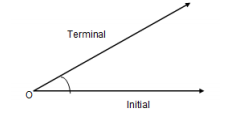2. If the direction of the rotation is anticlockwise, the angle is said to be positive and if the direction of the rotation is clockwise, then the angle is negative.3. If a rotation from the initial side to terminal side is (1/360)th of a revolution, the angle is said to have a measure of one degree, I t is denoted by 1o.

4. A degree is divided into 60 minutes, and a minute is divided into 60 seconds. One sixtieth of a degree is called a minute, written as 1’, and one sixtieth of a minute is called a second, written as 1”

Thus 1=60',1'=60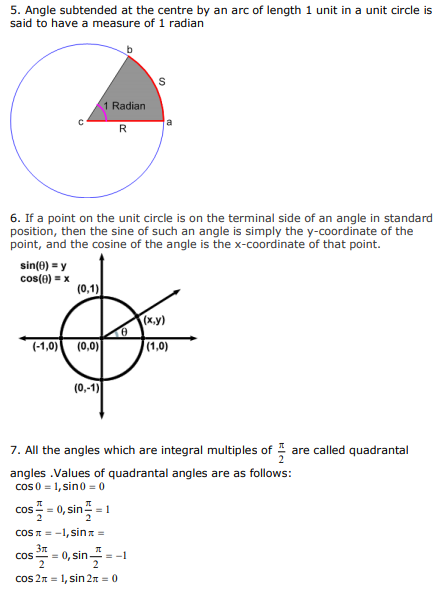8 .Cosine is even and sine is odd function
cos(-x) = cos x
sin(-x) = - sin x

9. Signs of Trigonometric functions in various quadrants

In quadrant I, all the trigonometric functions are positive.

In quadrant II, only sine is positive. In quadrant III, only tan is positive,

quadrant IV, only cosine function is positive. This is depicted as follows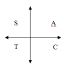10. In quadrants where Y-axis is positive (i.e. I and II), sine is positive and in quadrants where X-axis is positive (i.e. I and IV), cosine is positive

11. A function f is said to be a periodic function if there exists a real number T>0, such that f(x + T) = f(x) for all x. This T is the period of function.

12. sin (2π+ x ) = sin x so the period of sine is 2π. Period of its reciprocal is also 2π

13. cos (2π+ x) = cos x so the period of cos is 2π. Period of its reciprocal is also 2π

14. tan (π+ x) = tan x Period of tangent and cotangent function is π

15.The graph of cos x can be obtained by shifting the sin function by the factor 2π

16. The tan function differs from the previous two functions in two ways (i)tan is not defined at the odd multiples of π/2 (ii) tan function is not bounded.

17. Function Period
y=sin x            2π

y=sin (ax)       2π/a

y=cos x           2π

y=cos (ax)      2π/a

y=cos 3x        2π/3

y=sin 5x        2π/5

18. For a function of the form
y= kf(ax+b) range will be k times the range of function x, where k is any real number if f(x)= sine or cosine function range will be equal to R-[-k, k] if function is of the form sec x or cosec x, Period is equal to the period of function f by a. The position of the graph is b units to the right/left of y=f(x) depending on whether b>0 or b<0

19. The solutions of a trigonometric equation for which 0 ≤ x ≤ 2π are called principal solutions.

20.The expression involving integer ‘n’ which gives all solutions of a trigonometric equation is called the general solution.

21. The numerical smallest value of the angle (in degree or radian) satisfying a given trigonometric equation is called the Principal Value. If there are two values, one positive and the other negative, which are numerically equal,
then the positive value is taken as the Principal value.
Top Formulae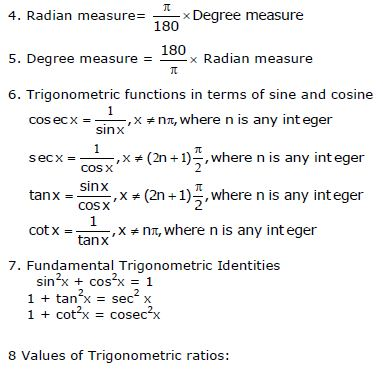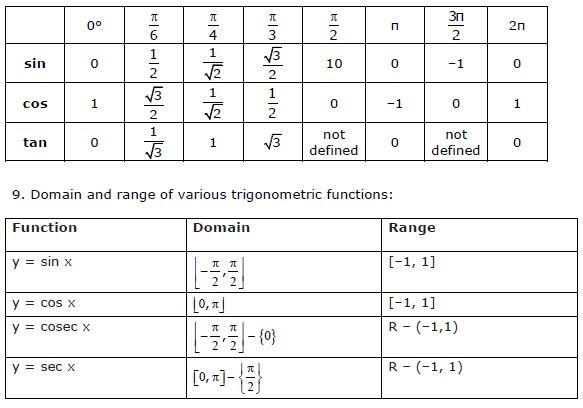CBSE Class 11 Mathematics Set Theory Notes
 CBSE Class 11 Mathematics Relations And Functions Notes
 CBSE Class 11 Mathematics Complex Numbers Quadratic Equations Notes
 CBSE Class 11 Mathematics Linear Inequalities Notes
 CBSE Class 11 Mathematics Permutation And Combination Formulas And Questions CBSE Class 11 Mathematics Permutation And Combination Notes
 CBSE Class 11 Mathematics Sequence And Series Formulas And Questions CBSE Class 11 Mathematics Sequence And Series Notes
 CBSE Class 11 Mathematics Straight Lines Formulas And Questions
 CBSE Class 11 Mathematics Coordinate Geometry Formulas And Questions CBSE Class 11 Mathematics Three Dimensional Geometry Notes
 CBSE Class 11 Mathematics Limits And Derivatives Notes
 CBSE Class 11 Mathematics Mathematical Reasoning Notes
 CBSE Class 11 Mathematics Statistics Notes
 CBSE Class 11 Mathematics Probability Notes

## More Study Material

### CBSE Class 11 Mathematics Chapter 3 Trigonometric Functions Notes

We hope you liked the above notes for topic Chapter 3 Trigonometric Functions which has been designed as per the latest syllabus for Class 11 Mathematics released by CBSE. Students of Class 11 should download and practice the above notes for Class 11 Mathematics regularly. All revision notes have been designed for Mathematics by referring to the most important topics which the students should learn to get better marks in examinations. Studiestoday is the best website for Class 11 students to download all latest study material.

### Notes for Mathematics CBSE Class 11 Chapter 3 Trigonometric Functions

Our team of expert teachers have referred to the NCERT book for Class 11 Mathematics to design the Mathematics Class 11 notes. If you read the concepts and revision notes for one chapter daily, students will get higher marks in Class 11 exams this year. Daily revision of Mathematics course notes and related study material will help you to have a better understanding of all concepts and also clear all your doubts. You can download all Revision notes for Class 11 Mathematics also from www.studiestoday.com absolutely free of cost in Pdf format. After reading the notes which have been developed as per the latest books also refer to the NCERT solutions for Class 11 Mathematics provided by our teachers

#### Chapter 3 Trigonometric Functions Notes for Mathematics CBSE Class 11

All revision class notes given above for Class 11 Mathematics have been developed as per the latest curriculum and books issued for the current academic year. The students of Class 11 can rest assured that the best teachers have designed the notes of Mathematics so that you are able to revise the entire syllabus if you download and read them carefully. We have also provided a lot of MCQ questions for Class 11 Mathematics in the notes so that you can learn the concepts and also solve questions relating to the topics. All study material for Class 11 Mathematics students have been given on studiestoday.

#### Chapter 3 Trigonometric Functions CBSE Class 11 Mathematics Notes

Regular notes reading helps to build a more comprehensive understanding of Chapter 3 Trigonometric Functions concepts. notes play a crucial role in understanding Chapter 3 Trigonometric Functions in CBSE Class 11. Students can download all the notes, worksheets, assignments, and practice papers of the same chapter in Class 11 Mathematics in Pdf format. You can print them or read them online on your computer or mobile.

#### Notes for CBSE Mathematics Class 11 Chapter 3 Trigonometric Functions

CBSE Class 11 Mathematics latest books have been used for writing the above notes. If you have exams then you should revise all concepts relating to Chapter 3 Trigonometric Functions by taking out a print and keeping them with you. We have also provided a lot of Worksheets for Class 11 Mathematics which you can use to further make yourself stronger in Mathematics

Where can I download latest CBSE Class 11 Mathematics Chapter 3 Trigonometric Functions notes

You can download notes for Class 11 Mathematics Chapter 3 Trigonometric Functions for latest academic session from StudiesToday.com

Can I download the Notes for Chapter 3 Trigonometric Functions Class 11 Mathematics in Pdf format

Yes, you can click on the link above and download notes PDFs for Class 11 Mathematics Chapter 3 Trigonometric Functions which you can use for daily revision

Are the revision notes available for Chapter 3 Trigonometric Functions Class 11 Mathematics for the latest CBSE academic session

Yes, the notes issued for Class 11 Mathematics Chapter 3 Trigonometric Functions have been made available here for latest CBSE session

How can I download the Chapter 3 Trigonometric Functions Class 11 Mathematics Notes pdf

You can easily access the link above and download the Class 11 Notes for Mathematics Chapter 3 Trigonometric Functions for each topic in Pdf

Is there any charge for the Class 11 Mathematics Chapter 3 Trigonometric Functions notes

There is no charge for the notes for CBSE Class 11 Mathematics Chapter 3 Trigonometric Functions, you can download everything free of charge

Which is the best online platform to find notes for Chapter 3 Trigonometric Functions Class 11 Mathematics

www.studiestoday.com is the best website from which you can download latest notes for Chapter 3 Trigonometric Functions Mathematics Class 11

Where can I find topic-wise notes for Class 11 Mathematics Chapter 3 Trigonometric Functions

Come to StudiesToday.com to get best quality topic wise notes for Class 11 Mathematics Chapter 3 Trigonometric Functions

Can I get latest Chapter 3 Trigonometric Functions Class 11 Mathematics revision notes as per CBSE syllabus

We have provided all notes for each topic of Class 11 Mathematics Chapter 3 Trigonometric Functions as per latest CBSE syllabus